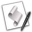## Filters

The following routines can be used to filter lists and text data.

### Highest Numeric Value in a List

This sub-routine will return the highest numeric value in a list of items. The passed list can contain non-numeric data as well as lists within lists. For example:

highest_number({-3.25, 23, 2345, "sid", 3, 67})
--> returns: 2345
highest_number({-3.25, 23, {23, 78695, "bob"}, 2345, true, "sid", 3, 67})
--> returns: 78695

If there is no numeric data in the passed list, the sub-routine will return a null string ("")

highest_number({"this", "list", "contains", "only", "text"})
--> returns: ""

Here's the sub-routine:A sub-routine that returns the highest value from the values passed to it:

on highest_number(values_list)
set the high_amount to ""
repeat with i from 1 to the count of the values_list
set this_item to item i of the values_list
set the item_class to the class of this_item
if the item_class is in {integer, real} then
if the high_amount is "" then
set the high_amount to this_item
else if this_item is greater than the high_amount then
set the high_amount to item i of the values_list
end if
else if the item_class is list then
set the high_value to highest_number(this_item)
if the the high_value is greater than the high_amount then ¬
set the high_amount to the high_value
end if
end repeat
return the high_amount
end highest_number

### Lowest Numeric Value in a List

This sub-routine will return the lowest numeric value in a list of items. The passed list can contain non-numeric data as well as lists within lists. For example:

lowest_number({-3.25, 23, 2345, "sid", 3, 67})
--> returns: -3.25
lowest_number({-3.25, 23, {-22, 78695, "bob"}, 2345, true, "sid", 3, 67})
--> returns: -22

If there is no numeric data in the passed list, the sub-routine will return a null string ("")

lowest_number({"this", "list", "contains", "only", "text"})
--> returns: ""

Here's the sub-routine:A sub-routine that returns the lowest numeric value from the numeric values passed to it:

on lowest_number(values_list)
set the low_amount to ""
repeat with i from 1 to the count of the values_list
set this_item to item i of the values_list
set the item_class to the class of this_item
if the item_class is in {integer, real} then
if the low_amount is "" then
set the low_amount to this_item
else if this_item is less than the low_amount then
set the low_amount to item i of the values_list
end if
else if the item_class is list then
set the low_value to lowest_number(this_item)
if the the low_value is less than the low_amount then ¬
set the low_amount to the low_value
end if
end repeat
return the low_amount
end lowest_number